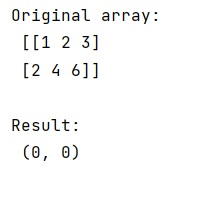# NumPy minimum in (row, column) format

Learn, how to find the index coordinates of the minimum values of a ndarray both in a row and a column?
Submitted by Pranit Sharma, on January 22, 2023

NumPy is an abbreviated form of Numerical Python. It is used for different types of scientific operations in python. Numpy is a vast library in python which is used for almost every kind of scientific or mathematical operation. It is itself an array which is a collection of various methods and functions for processing the arrays.

## Finding the index coordinates of the minimum values of a ndarray both in a row and a column

Suppose that we are given a 2-dimensional NumPy array and we need to find the (row, column) index of the minimum of a NumPy array.

For this purpose, we will use the unravel_index() method where we can use the argmin() method. This method converts a flat index or array of flat indices into a tuple of coordinate arrays.

Syntax: [Ref: numpy.unravel_index()

```numpy.unravel_index(indices, shape, order='C')
```

Parameter(s):

• indices: array_like - An integer array whose elements are indices into the flattened version of an array of dimensions shape. Before version 1.6.0, this function accepted just one index value.
• shape: tuple of ints - The shape of the array to use for unraveling indices.
• order: {'C', 'F'}, optional - Determines whether the indices should be viewed as indexing in row-major (C-style) or column-major (Fortran-style) order.

Let us understand with the help of an example,

## Python code to find the index coordinates of the minimum values of a ndarray both in a row and a column

```# Import numpy
import numpy as np

# Creating a numpy array
arr = np.array([[1,2,3],[2,4,6]])

# Display original array
print("Original array:\n",arr,"\n")

# Return the row and column index
# of minimum element
res = np.unravel_index(arr.argmin(), arr.shape)

# Display result
print("Result:\n",res,"\n")
```

Output: